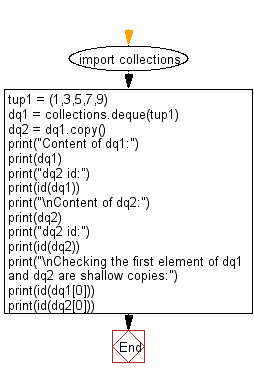﻿ Python: Copy of a deque object and verify the shallow copying process - w3resource# Python: Copy of a deque object and verify the shallow copying process

## Python Collections: Exercise-11 with Solution

Write a Python program to copy of a deque object and verify the shallow copying process.

Sample Solution:

Python Code:

``````import collections
tup1 = (1,3,5,7,9)
dq1 = collections.deque(tup1)
dq2 = dq1.copy()
print("Content of dq1:")
print(dq1)
print("dq2 id:")
print(id(dq1))
print("\nContent of dq2:")
print(dq2)
print("dq2 id:")
print(id(dq2))
print("\nChecking the first element of dq1 and dq2 are shallow copies:")
print(id(dq1))
print(id(dq2))
```
```

Sample Output:

```Content of dq1:
deque([1, 3, 5, 7, 9])
dq2 id:
140706429557152

Content of dq2:
deque([1, 3, 5, 7, 9])
dq2 id:
140706406914152

Checking the first element of dq1 and dq2 are shallow copies:
11065888
11065888
```

Flowchart:## Visualize Python code execution:

The following tool visualize what the computer is doing step-by-step as it executes the said program:

Python Code Editor:

Have another way to solve this solution? Contribute your code (and comments) through Disqus.

What is the difficulty level of this exercise?

Test your Programming skills with w3resource's quiz.

﻿

## Python: Tips of the Day

Use Reversed() In for Loops:

```>>> tasks = ['laundry', 'picking up kids', 'gardening', 'cooking']